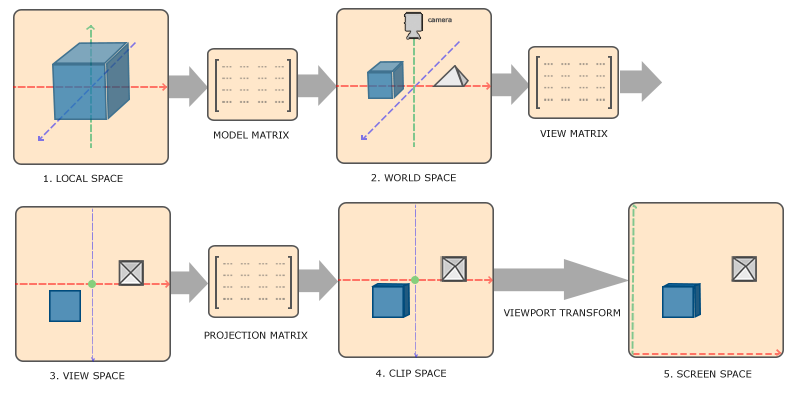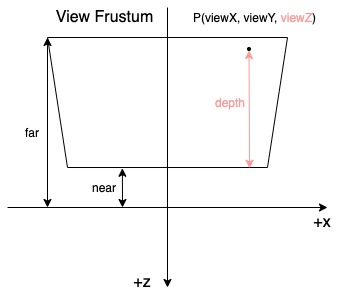# 空间变换1 2 3 4 5 6 7 8 9 10 11 12 13 14 15  attribute vec3 localPosition; // local space -> world space vec4 worldPosition = modelMatrix * vec4(localPosition, 1.0); // world space -> view space vec4 viewPosiion = viewMatrix * worldPosition; // view space -> clip space vec4 clipPosition = projectionMatrix * viewPosition; // clip space -> ndc space vec3 ndcPosition = clipPosition.xyz / clipPosition.w; // ndc space -> screen space gl_FragCoord = vec3( (ndcPosition.x + 1) * width / 2 + x, (ndcPosition.y + 1) * height / 2 + y, (ndcPosition.z + 1) * (far - near) / 2 + near );

# 投影变换

• 透视矩阵
$$\begin{bmatrix} {\frac {2n} {r-l}} & 0 & {\frac {r+l} {r-l}} & 0 \\ 0 & {\frac {2n} {t-b}} & {\frac {t+b} {t-b}} & 0 \\ 0 & 0 & {\frac {-(f + n)} {f - n}} & {\frac {-2fn} {f - n}} \\ 0 & 0 & -1 & 0 \end{bmatrix}$$
• 正交矩阵
$$\begin{bmatrix} {\frac {2} {r-l}} & 0 & 0 & -{\frac {r+l} {r-l}} \\ 0 & {\frac {2} {t-b}} & 0 & -{\frac {t+b} {t-b}} \\ 0 & 0 & {\frac {-2} {f-n}} & -{\frac {f+n} {f-n}} \\ 0 & 0 & 0 & 1 \end{bmatrix}$$

## 透视投影变换

$$W_{clip} = -Z_{view}$$ $$Z_{ndc} = \frac {Z_{clip}} {W_{clip}} = \frac {A Z_{view} + B} {-Z_{view}} = -A - \frac B {Z_{view}}$$

## 正交投影变换

$$W_{clip} = W_{view} = 1.0$$ $$Z_{ndc} = \frac {Z_{clip}} {W_{clip}} = A {Z_{view}} + B$$

# 深度与 View Z

View Z 指的是 view space 下的 Z 值，也就是刚刚一直提到的 $Z_{view}$。深度与View Z 的关系大概可以用下图来表示：• 当使用正交投影时，深度是线性变化的。
• 当使用透视投影时，深度是非线性变化的。

$$Z_{ndc} = A {Z_{view}} + B$$

$$Z_{ndc} = -A - \frac B {Z_{view}}$$

# 深度缓冲（Z Buffer）

$$Z_{buffer} = Z_{ndc} \times 0.5 + 0.5$$

## 从透视投影求 View Z

$\frac {2fn} {(f+n) - Z_{ndc}(f-n)}$

$\frac {fn} {f - Z_{buffer}(f-n)}$

## 从正交投影求 View Z

$n - Z_{buffer}(n - f)$

## 计算 view space 下的坐标

  1 2 3 4 5 6 7 8 9 10 11 12 13 14 15 16 17 18 19 20 21 22 23 24  // NOTE: 外部传递进来的变量 uniform float near; uniform float far; uniform mat4 projectionMatrix; uniform mat4 projectionInverseMatrix; uniform sampler2D depthMap; varying vec2 uv; void main() { // NOTE: 假设要绘制一个铺满屏幕的矩形，uv 刚好可以用来表示它的屏幕坐标，取值范围是 [0, 1] vec2 screenPosition = uv; float zBuffer = texture2D(depthMap, uv).r; // NOTE: 假设用透视投影 float viewZ = (far * near) / ((far - near) * zBuffer - far); float clipW = projectionMatrix * viewZ + projection; // NOTE: Az + B vec3 ndcPosition = vec3(screenPosition, zBuffer) * 2.0 - 1.0; vec4 clipPosition = vec4(ndcPosition, 1.0) * clipW; vec4 viewPosition = projectionInverseMatrix * clipPosition; // ... }

# 参考资料

Coordinate Systems

OpenGL Projection Matrix

Linear Depth